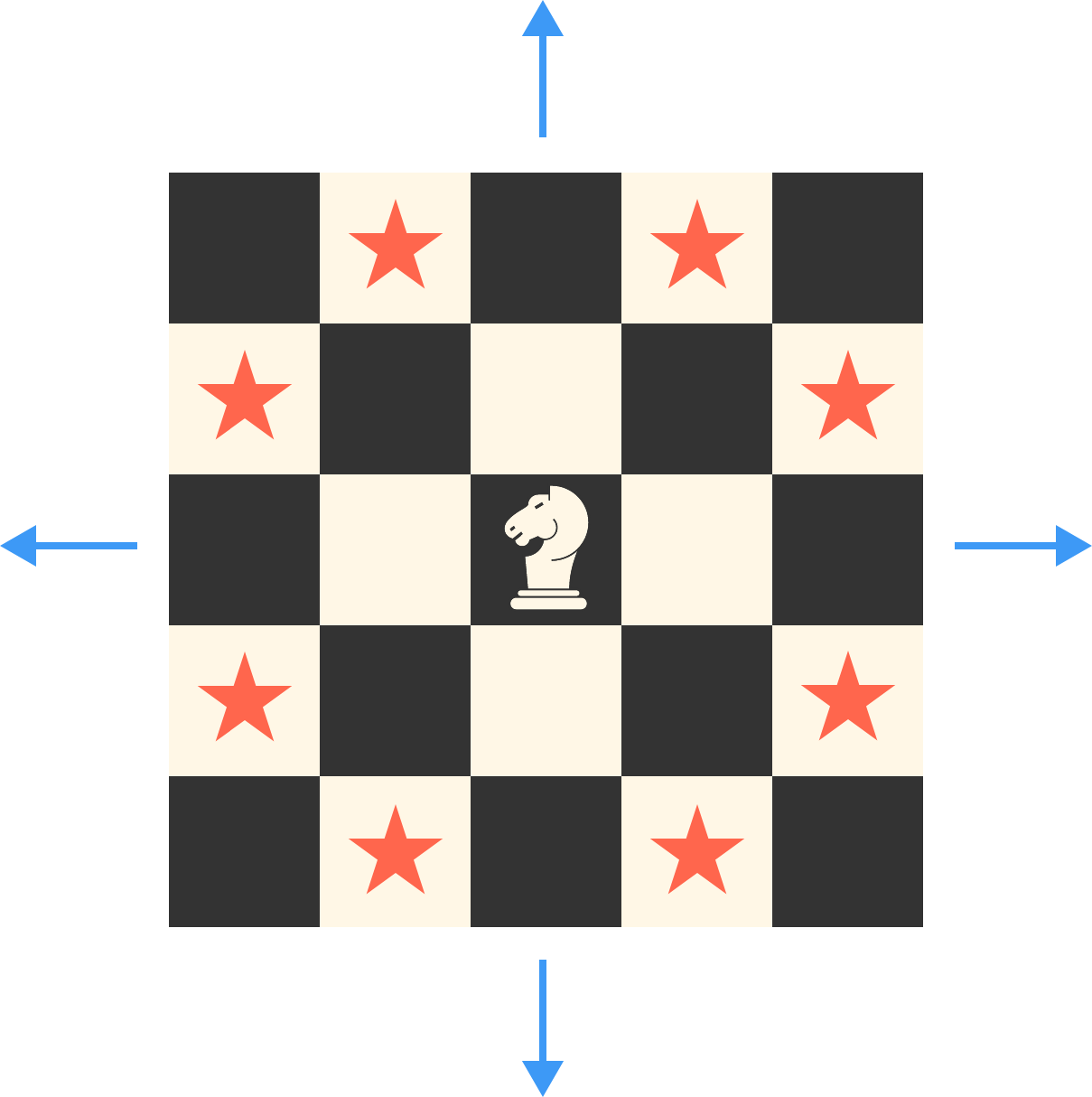# Problems of the Week

Contribute a problem

Consider a $2017 \times 2017$ square grid. Each cell is filled in with a real number with absolute value at most 1, such that the sum of any $2 \times 2$ square grid is exactly 0.

Let $S$ be the sum of all of these real numbers. What is the maximum value of $S$?

Note: Below is a $3 \times 3$ grid which satisfies the requirements above (although its sum is not necessarily maximized).

$\Large \begin{array}{|c|c|c|} \hline -0.3 & 0.2 & -0.6 \\ \hline 0.4 & -0.3 & 0.7 \\ \hline 0.5 & -0.6 & 0.2 \\ \hline \end{array}$

Let $f(x)$ be a polynomial function with integer coefficients such that $f(1) = 5, \quad f(2) = 8, \quad f(3) = 13.$

What is the minimum possible positive value of $f(21)$?

On the Cartesian plane, is it possible to place an uncountable number of figure 8's (two circles that are externally tangential) that do not intersect each other?


Note: An infinite set is uncountable if there exists no bijection to the integers.

Lazy Larry was assigned to create a bubble sort algorithm, but he didn't have the time to do it properly. He designed the following algorithm instead:

For each iteration of the algorithm:

$\vphantom{1}$

• Choose two distinct elements in the list uniformly at random.

• Exchange the placements of those elements in the list.

• Check to see if the list is now sorted. If it is, end the algorithm. Otherwise, do another iteration.

Lazy Larry tests his algorithm on the following list:

capybara, dingo, aardvark, bandicoot

What is the expected value of the number of iterations of Larry's algorithm to alphabetize the list (A to Z)?

A knight is placed at the center of an infinitely large chess board. For large $n,$ the number of squares on which the knight can be after exactly $n$ moves is asymptotically $a \cdot n^b$ for real numbers $a$ and $b.$ Find $a+b.$Note: A chess knight moves in an "L" shape either 1 square vertically and 2 squares horizontally or 2 squares vertically and 1 square horizontally, as indicated by the stars above.

×